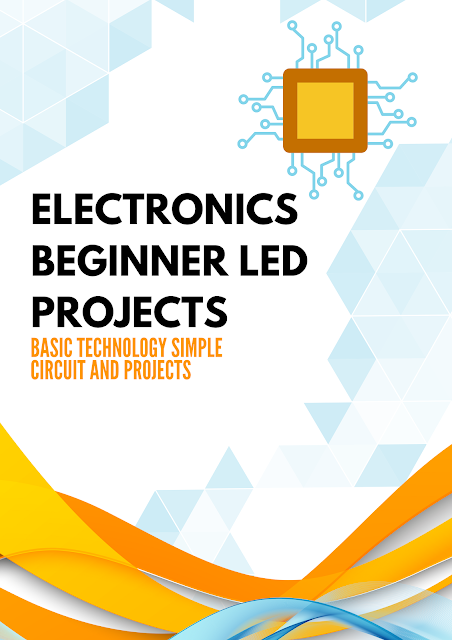# Electronics Led Projects : The Basic Technology Simple Circuit and Projects ..Guide for beginners STEP BY STEP

##Electronics is a science that studies electronic chips and circuits of electronic devices and their operating principle. "It depends mainly on the study of the flow of electrical (electronic) charges and their circulation through media such as; Air, gases and semiconductors.

The world of electronics is a world full of fun, energy and control over everything through electronic and electrical circuits. What is the basic structure of any electronic device, invention or design. Your understanding of electronic circuits is the first step to entering the world of electronics, analyzing it and understanding how it works and where to start working.

And because any electronic circuit is made up of a group of elements; These are the electronic elements, so you need to understand the functionality of electronic components and analyze their circuits. We have provided you with a comprehensive guide to start your learning in the field of electronics.

Circuit Analysis is a fundamental aspect of electrical engineering that deals with the study of electrical circuits and their behavior. It involves determining the flow of electric current, voltages, and power in a circuit and is a crucial aspect of designing and optimizing electrical systems.

One of the primary tools used in circuit analysis is Ohm's Law, which states that the current flowing through a conductor between two points is directly proportional to the voltage across the two points and inversely proportional to the resistance between them. This relationship can be represented mathematically as V=IR, where V is the voltage, I is the current, and R is the resistance.

Circuit analysis can be broken down into two main categories: DC (direct current) analysis and AC (alternating current) analysis. In DC analysis, the circuit is analyzed under steady-state conditions, where the current and voltage in the circuit remain constant over time. In AC analysis, the circuit is analyzed under dynamic conditions, where the current and voltage change over time.

One of the most common methods of circuit analysis is nodal analysis, also known as Kirchhoff's current law. This method involves identifying all the nodes in the circuit and writing equations for the current flowing into and out of each node. The equations are then solved to determine the unknown currents and voltages in the circuit.

Another important method of circuit analysis is mesh analysis, also known as Kirchhoff's voltage law. This method involves identifying all the loops in the circuit and writing equations for the voltage drop around each loop. The equations are then solved to determine the unknown currents and voltages in the circuit.

Circuit analysis can also be performed using simulation tools, such as PSpice, LTSpice, Proteus, MATLAB, and Multisim. These tools allow engineers to model and simulate circuits in a virtual environment, without the need for physical prototypes. The simulation results can then be used to determine the performance of the circuit, identify potential issues, and optimize the design.

One of the advantages of using simulation tools for circuit analysis is the ability to test different design scenarios quickly and easily. For example, an engineer can simulate the impact of changing component values, adding or removing components, or varying the load on the circuit. This allows engineers to make informed design decisions and improve the performance of the circuit.

In conclusion, circuit analysis is an essential aspect of electrical engineering that deals with the study of electrical circuits and their behavior. It involves determining the flow of electric current, voltages, and power in a circuit and is a crucial aspect of designing and optimizing electrical systems. Circuit analysis can be performed using mathematical methods, such as nodal and mesh analysis, or using simulation tools, such as PSpice, LTSpice, Proteus, MATLAB, and Multisim. By using these tools and techniques, engineers can improve the efficiency and accuracy of their circuit analysis and design processes.

A Electronics Beginner Led Projects : The Basic Technology Simple Circuit and Projects ..Guide for beginners STEP BY STEP .pdf